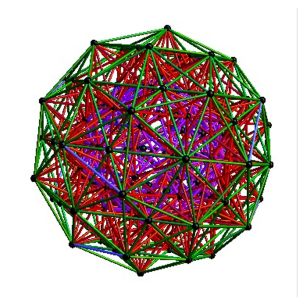# Dual 600 Cell (H4+H4/Phi) Petrie Projection to Square,Penta & Hexagon

I have published my ToE.pdf to vixra.org http://vixra.org/abs/1006.0063 .  While looking around vixra, I found a paper by Tony Smith on E8 http://vixra.org/pdf/0907.0006v1.pdf.  It has much of the work on his website including references to Lisi and Bathsheba on their visualizations of E8.  Some of the references are based on the 600 Cell set H4 & H4/Phi (shown by Richter to be isomorphic to E8). I can show the E8 Dynkin diagram can indeed be folded to H4.

The H4 and its 120 vertices make up the 4D 600 Cell (which is made up of 96 vertices of the snub-24 Cell and the 24 vertices of the 24 Cell=[16 vertex Tesseract=8-Cell and the 8 vertices of the 4-Orthoplex=16 Cell]). It can be generated from the 240 split real even E8 vertices using a 4×8 rotation matrix:
x = (1, φ, 0, -1, φ, 0, 0, 0)
y = (φ, 0, 1, φ, 0, -1, 0, 0)
z = (0, 1, φ, 0, -1, φ, 0, 0)
w = (0, 0, 0, 0, 0, 0, φ^2, 1/φ)

where φ=Golden Ratio=(1+Sqrt(5))/2

I find in folding from 8D to 4D, that the 6720 edge counts split into two sets of 3360 from E8’s 6720 length Sqrt(2).

I recreated here a E8 Petrie projection which is isomorphic to 2D split real even E8 Petrie projection (except it only produces half of the 6720 “shortest edge” counts, of different length). All of these use a single set of 3 projection basis vectors to produce a single 3D projected object with 3 unique faces. On one face, it has the famous E8 Petrie projection:

On another face it has a pentagonal projection:

On the third unique face is the hexagonal projection:

I created a movie (similar to those of Lisi) of the H4+H4/Phi 600 Cells that rotates the 2D H-V E8 face projection to the H-Z Pentagonal face (shown in the middle frames of the movie). This is an interesting rotation because this rotates through a square projection at around Pi/8.

A lower quality YouTube is here: 600 Cells E8 to Pentagonal.  Download and play the higher quality .avi: cell600E8pent

I also created a 3D perspective spin of that projection (two sets of mutually orthogonal cubic faces). On YouTube:600 Cells 3D Spin.  Download the higher quality .avi: cell6003Dspin2

Similar to the work of other 3D artists like Bathsheba and Wizzy in Second Life (SL), this object can be created in Real Life (RL) as 3D laser etched crystal or rezzed as a 3000+ prim object in SL.

The 3 (H,V,Z) basis projection vectors are: